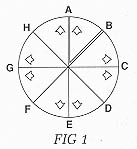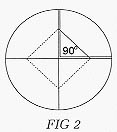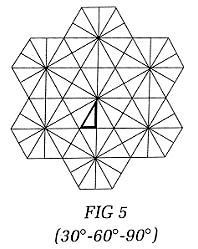Kaleidoscope Mirror Arrangements by Charles Karadimos There are 2 basic systems of mirrors in kaleidoscopes, the 2-mirror which produces one central image and the 3-mirror which produces images reflected throughout the entire field of view. Both are set up in a triangular configuration-in a tube similar to a prism. Considering the 2-mirror system first, the 2 mirrors are arranged in a "V" with the third side of the triangle a blackened, non-reflective surface. The angle of the "V" determines the number of reflections contributing to the overall intricacy of the pattern. In an attempt to show what happens in a kaleidoscope consider the following example.Circle with center 0-Mirrors OA and OB. An object placed in Triangle OAB (actual image) is reflected off OA into its relative position in Triangle OHA (virtual image) and off OB into its relative position in Triangle OBC. The virtual image in Triangle OHA is reflected off the imaginary OH into its relative position in Triangle OGH - which is reflected off OG into its relative position in Triangle OFG. This continues the same way around the circle from Triangle OBC to Triangle OCD to Triangle ODE. The virtual image in Triangle OEF is a reflection from the combination of OF and OE. In order for the symmetry to be adhered to, it is critical that the angle AOB be such that it evenly divides the 360o of the circle. If it does not, then the reflections from OF and OE in Triangle OEF would not correspond. An inaccurate overlap would inherently destroy the closure of the symmetric pattern. Therefore a basic rule of thumb in a 2-mirror scope is that the angle of the mirrors must evenly divide the 360o of a circle.So, starting with a 90 degree angle, the image produced would have 4 fold symmetry (FIG 2). 45 degree - 8 fold symmetry - 4 point star 36 degree - 10 fold symmetry - 5 point star 30 degree - 12 fold symmetry - 6 point star 22.5 degree - 16 fold symmetry - 8 point star 15 degree - 24 fold symmetry - 12 point star 10 degree - 36 fold symmetry - 18 point star 1 degree - 360 fold symmetry - 180 point star The 3-mirror system reacts similarly to the 2-mirror with one major exception. A third mirror replaces the blackened side of the triangle in the 2-mirror and produces a continuation of reflections throughout the entire field of view. Symmetrical images are much harder to achieve in 3-mirror systems because now there are 3 angles which must be accurate instead of only the one angle in the 2-mirror design. Again it is important that the mirrors be set at an angle which can be evenly divided into the 360 degrees of the circle; such as 90 degree, which divides into 360 degrees 4 times, or: 60 degree - which divides 6 times 45 degree - which divides 8 times 36 degree - which divides 10 times 30 degree - which divides 12 times The other important rule which governs symmetry throughout is that the sum of the 3 angles must total 180 degrees (the total number of degrees in a triangle). Using both these rules, only 3 combinations produce the desired effect. The most common and simplest arrangement is the 60 - 60 - 60 equilateral triangle. Here each angle produces 6 fold patterns which results in a design (FIG3) of continuous triangles.The second combination is the 45 - 45 - 90 isosceles right triangle. This relationship produces 8 fold patterns at the 45 degree angles and a 4 fold pattern at the 90 degree angle, effectively producing continuous square patterns (FIG 4).The third and most interesting is the 3O - 60 - 90 right triangle. In this case all 3 angles are different, thus enabling 3 different symmetries (30 degree - 12 fold; 60 degree - 6 fold; 90 degree - 4 fold) to combine and form patterns as in FIG 5.One added note for 3-mirror configurations: the systems discussed above consider pure symmetrical patterns where each image - actual & virtual - link together. Interesting patterns are achieved however, even if only one or two of the angles follow the rule of even divisibility into 360o. The resulting pattern remains visibly symmetric. However, the continuing design generated by the odd angle or angles only show fractional parts of the actual image. Other systems, such as square 4-mirror configurations produce repeated square patterns while 4-mirror rectangular configurations produce repeated rectangular patterns. The images created are striped patterns since the reflections move directionally up, down, right and left. Cylindrical tubes lined with a reflective material will produce a spiralling effect. Since there are no angles involved in this style, the reflection seems to climb through the tube asymmetrically. Tapered systems provide interesting results. For instance, by tapering the 3-mirror triangle (similar to a pyramid) and viewing through the large opening, a spherical 3-D appearance results. Viewing through the small opening, the actual and virtual images are enlarged to more visibly examine the objects in the object chamber. This also allows more light to enter the system, increasing the overall brightness. The polyangular arrangement is a variation of the 2-mirror design, whereby one or both of the mirrors can be adjusted, changing the angle of the "V" and thus the number of reflections. Within the same tube it is therefore possible to produce a wide range of symmetrical patterns. Even though other systems and configurations are quite possible, these basic formulas should help in the understanding of the various principles underlying kaleidoscope design and the great variations possible in the images which can be created.